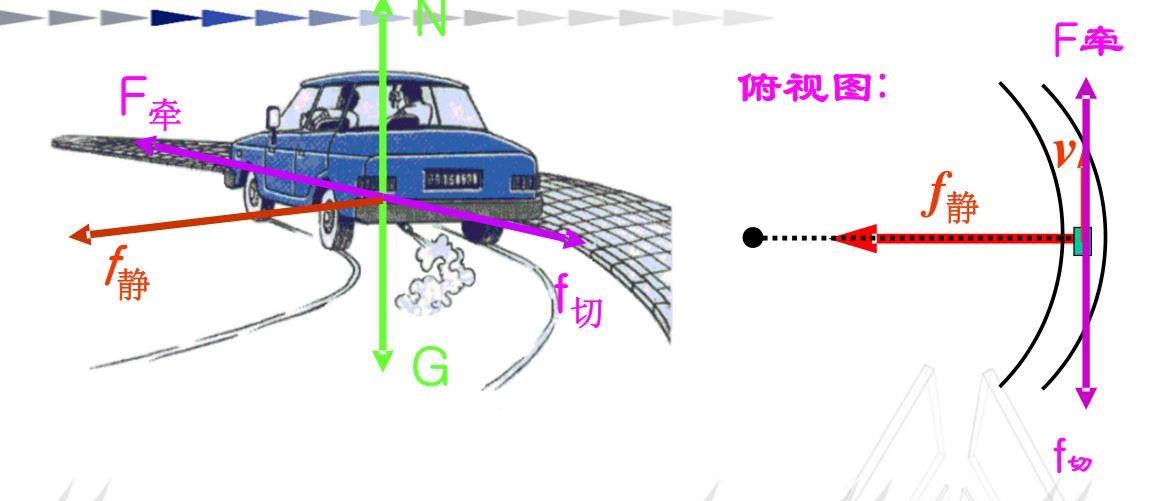llo：第16届智能车智能视觉组-上海交通大学AuTop战队开源汇总

一. 速度控制方案

1. 1. 常规速度控制float radius_3pts(float pt0, float pt1, float pt2){
float a, b, c, d, e, f, r, x, y;
a = 2*(pt1-pt0);
b = 2*(pt1-pt0);
c = pt1*pt1+pt1*pt1-pt0*pt0-pt0*pt0;
d = 2*(pt2-pt1);
e = 2*(pt2-pt1);
f = pt2*pt2+pt2*pt2-pt1*pt1-pt1*pt1;
x = (b*f-e*c)/(b*d-e*a);
y = (d*c-a*f)/(b*d-e*a);
r = sqrt((x-pt0)*(x-pt0)+(y-pt0)*(y-pt0));
return r;
}

1. 2. 特殊元素速度控制

1. 3.多模态PID切换

二．差速标定方法

C车模采用的是阿克曼结构，特点是，对于车模来说，小车在转向时四轮的垂线交于一点。依据阿克曼转向几何设计的车辆，沿着弯道转弯时，利用四连杆的相等曲柄使内侧轮的转向角比外侧轮大大约2-4度，使四个轮子路径的圆心大致上交会于后轴的延长线上瞬时转向中心，让车辆可以顺畅的转弯

1.前束与外倾

2.轮胎

SJTU-AuTop完整开源方案链接，如果觉得我们的方案对您有帮助，请在github上帮忙点个star吧:)

llo：第16届智能车智能视觉组-上海交通大学AuTop战队开源汇总